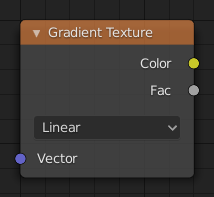The Gradient Texture node generates interpolated color and intensity values based on the input vector.

## Inputs¶

Vector

Texture coordinate to sample texture at; defaults to Generated texture coordinates if the socket is left unconnected.

## Properties¶

Type

Controls the type of gradient generated.

Linear

Directly returns the input X coordinate.

Interpolates the input X coordinate quadratically.

Easing

Uses a combination of quadratic and linear interpolation to return a smooth gradient from the input X coordinate.

Diagonal

Averages the input X and Y coordinates.

Spherical

Creates an inverse gradient using the length of the input vector; the maximum value is at (0, 0, 0).

The same as Spherical, except interpolated quadratically.

Returns a value based on the angle of the input around the Z axis.

## Outputs¶

Color

Texture color output.

Factor

Texture intensity output.